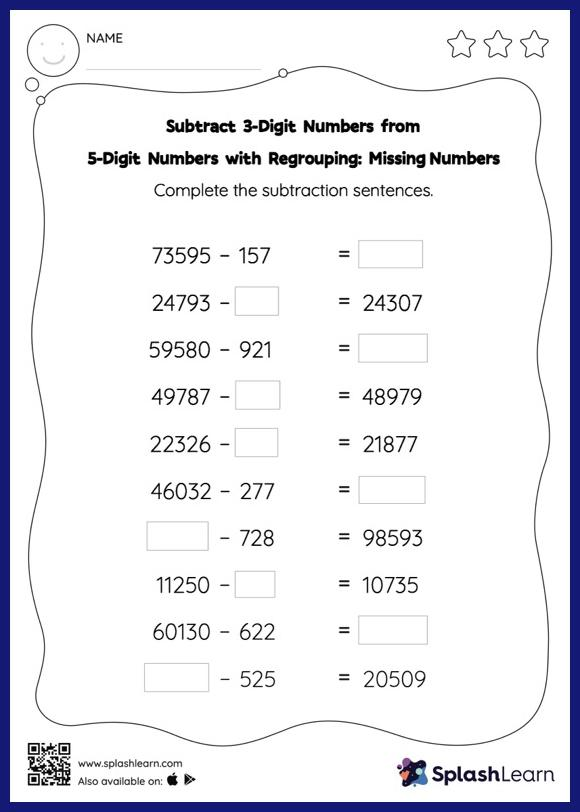# Subtract 3-Digit Numbers from 5-Digit Numbers with Regrouping: Missing Numbers Worksheet

Home > Subtract 3-Digit Numbers from 5-Digit Numbers with Regrouping: Missing NumbersSubtract 3-digit numbers from 5-digit numbers with regrouping worksheet helps students develop proficiency with subtraction. Students use the count back strategy or the relationship between addition and subtraction to find the missing number in this worksheet. They also need to regroup numbers to get to the answer in subtract 3-digit numbers from 5-digit numbers with regrouping worksheet. This worksheet is about practicing with the horizontal format in which numbers are written side by side. To develop flexibility with numbers and operations, students need to have enough practice in this format and not just rely on the vertical/column method.